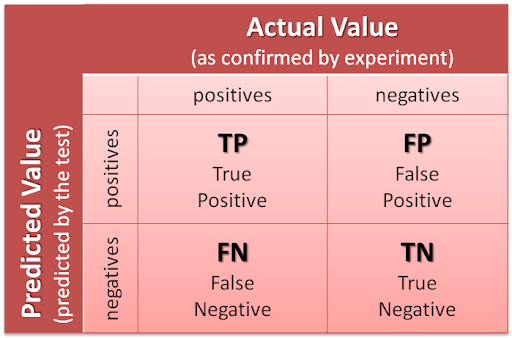# Performance Evaluation Parameters of a Machine Learning ModelThis gives a good idea of how our model performs it not only tells us how many classifications are done are correctly and how many of them are classified incorrectly but it tells more.

Understanding Various methods used to measure the performance of a Machine Learning Model.It is very Important to understand thoose to further improve on the aspect that is best suited for our Problem Statement.

So let us explore………

## Confusion Matrix

As the name suggests the Confusion Matrix is really confusing but let me break it down for you and explain it.Confusion Matrix is one of the most popular and most used Evaluation Parameters used in Classification Problems. This gives a good idea of how our model performs it not only tells us how many classifications are done are correctly and how many of them are classified incorrectly but it tells more.So here we have 2 rows positive and negative corresponding to predicted values and 2 columns positive and negative corresponding to actual values.

So we get a 2*2 matrix which has four positions to be filled let us describe all four of them.

1] True Positive-These are the samples which are correctly classified. They were True or 1 and they were predicted as 1

Eg — Telling a pregnant women that she is pregnant

2] True Negative-These are the samples which are correctly classified. They were False or 0 and they were predicted as 0

Eg — Telling a man that he is not pregnant

## Most popular Data Science and Machine Learning courses — July 2020

Most popular Data Science and Machine Learning courses — August 2020. This list was last updated in August 2020 — and will be updated regularly so as to keep it relevant

## 8 Machine Learning Resources to Learn Data Structures and Algorithms

8 Machine Learning Resources to Learn Data Structures and Algorithms. Before we start, most of these machine learning resources do assume a basic knowledge of at least one programming language. You don’t need to be an expert by any means! You just need the programming basics. If you have never written code before, you may want to bookmark this article and return to it later.

## 15 Machine Learning and Data Science Project Ideas with Datasets

Learning is a new fun in the field of Machine Learning and Data Science. In this article, we’ll be discussing 15 machine learning and data science projects.

## Artificial Intelligence (AI) vs Machine Learning vs Deep Learning vs Data Science

Artificial Intelligence (AI) vs Machine Learning vs Deep Learning vs Data Science: Artificial intelligence is a field where set of techniques are used to make computers as smart as humans. Machine learning is a sub domain of artificial intelligence where set of statistical and neural network based algorithms are used for training a computer in doing a smart task. Deep learning is all about neural networks. Deep learning is considered to be a sub field of machine learning. Pytorch and Tensorflow are two popular frameworks that can be used in doing deep learning.

## Pipelines in Machine Learning | Data Science | Machine Learning | Python

Machine Learning Pipelines performs a complete workflow with an ordered sequence of the process involved in a Machine Learning task. The Pipelines can also